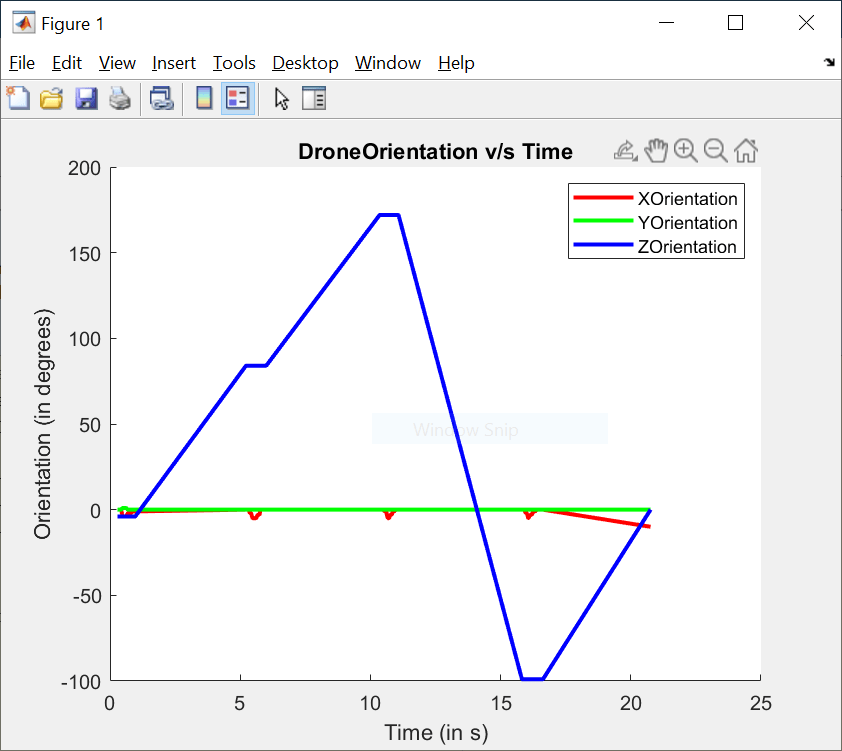Main Content

# Read and Plot Navigation data using MATLAB® Support Package for Ryze® Tello Drones

This example shows how to use the MATLAB® Support Package for Ryze® Tello Drones to acquire and plot the real-time navigation data of the Ryze® drone

### Introduction

The MATLAB Support Package for Ryze Tello Drones enables you to control and read the in flight navigation data of the drone.

In this example, you will learn to read navigation data of the Ryze drone such as the speed, orientation, and height using MATLAB commands.

### Required Hardware

To run this example you need the following:

• A fully charged Ryze Tello drone

• A computer with a WiFi connection

### Task 1 — Hardware setup

• Power on the Ryze drone.

• Connect your computer to the drone's Wifi network.

### Task 2 — Create a ryze object

Create a `ryze` object.

` r = ryze();`

### Task 3 — Take-off the drone

Start the Ryze drone flight from a level surface.

Execute the following command at the MATLAB command prompt the takeoff of the drone.

` takeoff(r);`

### Task 3 — Initialize MATLAB `animatedline` and `figure` window properties

This task shows you how to generate an animated plot for the orientation data.

Use MATLAB `animatedline` function to plot the variation in drone orientation along the X, Y, and Z axes, separately.

Initialize the figure handle and create animated line instances hx, hy, and hz corresponding to the orientation along the X, Y, and Z axes respectively.

``` f = figure; hx = animatedline('Color', 'r', 'LineWidth', 2); hy = animatedline('Color', 'g', 'LineWidth', 2); hz = animatedline('Color', 'b', 'LineWidth', 2); title('DroneOrientation v/s Time'); xlabel('Time (in s)'); ylabel('Orientation (in degrees)'); legend('XOrientation', 'YOrientation', 'ZOrientation');```

### Task 4 — Plot Navigation data during drone flight

Keep flying the drone along a square path and plot orientation data during this flight.

``` edgeIndex = 1; distance = 0.5; speed = 0.5; tObj = tic; while(edgeIndex <= 4) % Move the drone unblocking the command line tplot = tic; moveforward(r, 'Distance', distance, 'Speed', speed, 'WaitUntilDone', false); % Plot orientation while drone is moving while(toc(tplot)<distance/speed) orientation = rad2deg(readOrientation(r)); tStamp = toc(tObj); addpoints(hx, tStamp, orientation(2)); addpoints(hy, tStamp, orientation(3)); addpoints(hz, tStamp, orientation(1)); drawnow; end % Turn the drone after it has traversed one side of the square path pause(2); turn(r, deg2rad(90));```
``` edgeIndex = edgeIndex+1; end```

### Task 5 — Land the drone

Plot the final orientation and land the drone.

``` orientation = rad2deg(readOrientation(r)); tStamp = toc(tObj); addpoints(hx, tStamp, orientation(2)); addpoints(hy, tStamp, orientation(3)); addpoints(hz, tStamp, orientation(1)); drawnow; land(r);```### Task 6 — Clean up

When finished, clear the connection to the Ryze drone.

` clear r;`

Download ebook